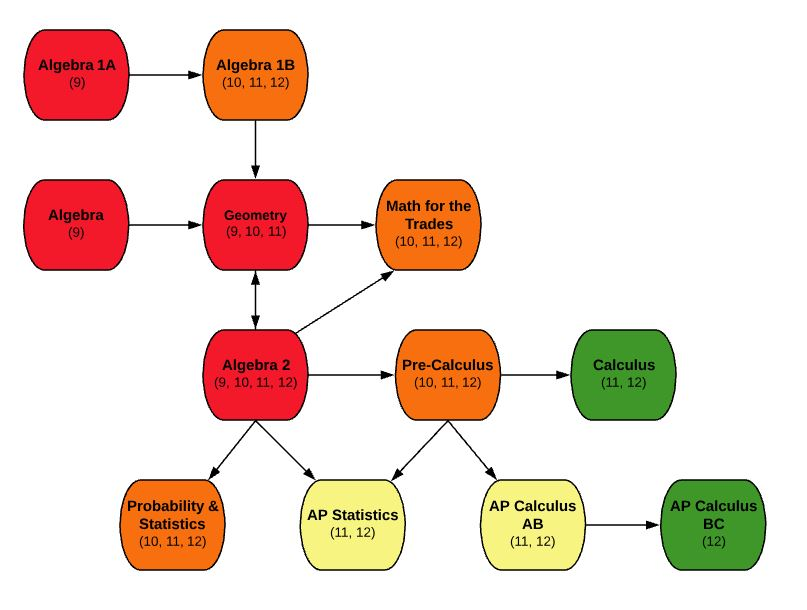EDIT MAIN

# MA400 Calculus12

Mathematics

Accelerated

0.5

This is a year long course that requires selection of both semesters: S1 and S2. Calculus prepares students for more advanced study in mathematics, science and other fields. The course begins with an extensive study of limits and uses this concept to develop differentiation and integration of polynomial, trigonometric, and logarithmic functions. A study is conducted in the application areas of science and economics using differentiation and integration. This course will cover most topics found in first semester college course, but at a slower pace and not as in depth as Advanced Placement Calculus. Students are required to have a TI 83 or comparable calculator.

Outcomes:

• Calculate limits graphically, algebraically, and numerically
• Find horizontal and vertical asymptotes
• Analyze continuity of a function
• Analyze instantaneous and average rate of change
• Understand the link between derivatives and instantaneous rate of change
• Use the appropriate method to calculate a derivative
• Use derivatives to calculate real world rates of change and apply these derivatives appropriately
• Use derivatives to analyze a function
• Estimate the area under the curve with finite sums
• Understand the connection between antiderivatives and integrals
• Use the appropriate method to calculate an antiderivative
• Use antiderivatives to calculate volume and other applications

MA414-S1 – Pre-Calculus S1 AND MA414-S2 – Pre-Calculus S2.

Students are required to have a TI 83 or comparable calculator.